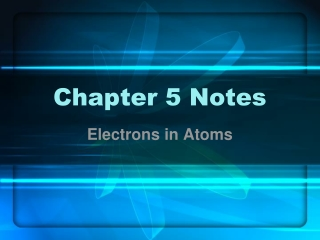DownloadDownload PresentationChapter 5 Notes

# Chapter 5 Notes

Télécharger la présentation## Chapter 5 Notes

- - - - - - - - - - - - - - - - - - - - - - - - - - - E N D - - - - - - - - - - - - - - - - - - - - - - - - - - -
##### Presentation Transcript

1. Chapter 5 Notes Electrons in Atoms

2. 5.1 Light and Quantized Energy • Rutherford’s nuclear model lacking • didn’t account for electron arrangement • couldn’t explain why diff. elements behave diff. • Elements emit visible light when heated in a flame

3. Wave Nature of Light • Electromagnetic radiation – form of energy that exhibits wavelike behaviors as it travels through space (visible light, microwaves, X-rays) • Speed • Wavelength (λ) – distance b/t two crests or troughs • Frequency (ƒ) – number of waves that pass a given point in one second; measured in Hz • Amplitude – height of wave from origin to crest or trough

4. Electromagnetic Spectrum • Encompasses all forms of electromagnetic radiation

5. Particle Nature of Light • Max Planck • Explained emission of light by heated objects (red hot ) • Quantum – minimum amount of energy gained/lost by an atom • Equantum = h • Photoelectric effect (explained by Einstein) • Photoelectrons emitted from metal’s surface • Electromagnetic radiation is both wavelike & particle - like • Photon – particle of electromagnetic radiation w/ no mass that carries a quantum of energy

6. Atomic Emission Spectra • Set of frequencies of the electromagnetic waves emitted by atoms of an element • An element’s “fingerprint”

7. 5.2 Quantum Theory and the Atom • Neils Bohr • Danish physicist • Proposed quantum model that explained why emission spectra were discontinuous

8. Bohr Model of the Atom • Ground state – lowest allowable energy state of an atom • Electrons orbit the nucleus in certain energy levels (circular orbits) • Smaller the orbit → lower the energy, & vice versa • Excited state – electrons move to higher energy levels when the atom gains energy • Photon emitted when electrons falls back to ground state

9. Quantum Mechanical Model • Bohr model determined incorrect • Only worked for hydrogen • Did not account for chemical behavior • Louis deBroglie proposed electron wave-particle duality • All moving particles exhibit wave-like characteristics • Heisenberg Uncertainty Principle – it is impossible to know both the velocity and position of a particle at the same time (He balloon)

10. Quantum Model… • Erwin Shrödinger – Austrian physicist; using complex equation, developed quantum mechanical model (electron cloud) of the atom • Electrons treated as waves • Electrons not in circular orbits • Mathematically predicts probable location of an electron

11. Quantum numbers • Every electron designated 4 quantum numbers • Principal quantum number (n): energy level • Sublevel (s, p, d, f) • Orbital – 3D region around nucleus that describes electron’s probable location • Spin • Diff. orbitals have diff. shapes

12. Orbital Shapes

13. Electron configuration • Arrangement of electrons in an atom • Aufbau principle – each electron occupies the lowest energy orbital available • All orbitals w/i an energy level are of equal energy (ie: 2px = 2py = 2pz, etc.) • All sublevels w/i an energy level have diff. energies (ie 2p > 2s, etc.) • Sublevels increase in energy according to s < p < d < f. • Orbitals of one sublevel may overlap those in another energy level.

14. Orbital filling…

15. Electrons Filling…

16. Write electron configurations for the first 10 elements.

17. Pauli exclusion principle – only two electrons with opposite spins can occupy an orbital Hund’s rule – single electrons with the same spin must occupy each equal energy orbital before electrons can be paired How to… Draw line for each orbital s: 1 p: 3 d: 5 f: 7 Draw arrow for each electron Follow Pauli’s & Hund’s rules Orbital Notation

18. What element is it?

19. Electron Dot Formulas • Valence electron – electron in an atom’s highest energy level • Identified by an element’s group number • No element has more than eight • Transition metals have either 1 or 2

20. Electron Dot Formulas… • Draw one dot for each valence electron • 1 5 8 2 4 6 7 3